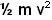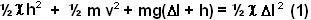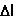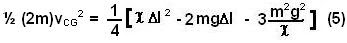## Sunday, May 11, 2008

### Irodov Problem 1.153As soon as the spring is released it pushes the mass B upwards. When B moves high enough, it will stretch the spring causing it to pull mass A upwards. Finally when spring is stretched enough, it will have enough tension to overcome mass A weight and lift it up. The problem can be solved easily with conservation of energy.

Suppose that the mass B, at some instant, is at a height h above the relaxed (un-stretched) length of the spring as shown in the figure. Suppose that the vertical velocity of B is v at this instant and mass A has not left the ground yet. Then the total energy of the system is the sum of i) the potential energy stored in the spring due to its extension given by, ii) the kinetic energy of mass B given byand iii) the potential energy gained by mass B during its motion upwards given by(since it has gained a height ofsince its initial position when the thread was intact). The initial energy content of the system is only that stored in the compressed spring given by. Since energy is conserved we have,There are three forces acting on mass A, i) the force of gravity mg pulling it down, ii) the tension in the springand iii) the normal reaction from the ground N. Since mass A is resting on the ground it has no acceleration.When, mass A is about to leave the ground, the normal reaction becomes 0 and so we have,a) When the initial compression in the springis just enough for mass A to leave the ground, the maximum height to which mass B will rise will be equal to the extension needed in the spring to lift A off the ground given by (3). At its highest point, mass B will have its velocity v=0 in (1).
So using this condition and substituting (3) in (1) we have,Ignoring the negative possibility we have,b) If the initial compression is, mass B will still be moving upwards when mass A lifts off the ground (at) , i.e. v>0. We can compute this vertical velocity v by using equation (1) as,The velocity of the center of mass of the system when mass A is about to leave the ground is given by,The kinetic energy of the CG when mass A is about to leave the ground is given thus by,Before mass A has left the ground, if mass B moves x units upwards, the CG will move x/2 units upwards. By the time mass A is about to leave the ground, mass B has movedup, so the CG would have moved up a height of. The total energy of the CG just before mass A leaves the ground is sum of its kinetic energy and its potential energy and is thus given by,This energy ECG must be conserved at all time. When the CG reaches its maximum height, its velocity will be zero. Suppose that the maximum height the CG attains is H, then it would have gained a potential energy of 2mgH and kinetic energy will be 0. So applying conservation of energy we have,Ifthen.

Abhinav Deshpande said...

thanks a lot !! I had not considered my system properly- the solution gave me some insight..Anonymous said...

nice solution!Ankur Sharma said...

excellent work!i ws not doing it in frame of centre of gravity and thus had 2 variables and one equation.Anonymous said...

pls clear my doubt.initially spring is compressed.then spring expands upper mass gains velocity and so do lower mass.and also moves upwards.then spring attains natural length but blocka still have upward velocity.so i believe when max ht is reached,blocks at rest there must be some compression in spring.in that case finall energy also must have spring pot. energy

Krishna Kant Chintalapudi said...

There will not only be spring compression at the highest point but also the masses could be moving towards or away from each other relative to the C.G (so that velocity of C.G is zero).

When you work with the C.G however, you think of the entire system (two masses + spring) as a point mass - the kinetic/potential energy of individual components of the system need not be accounted for.Anonymous said...

very nice solution. never occurred to me to solve from COM frame.
what would you do if the problem asked to find the max height attained by the upper block?